Simple Finite Heat Release Model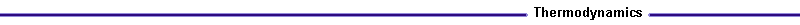Introduction We now account for finite burn duration, in which combustion occurs at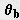, and continues until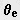. Figure 6 is a representation of this cycle. The peak pressure will not be as high as the Otto cycle which has a "delta" function heat release. The finite heat release model assumes that the heat input Qin is delivered to the cylinder over a finite crank angle duration.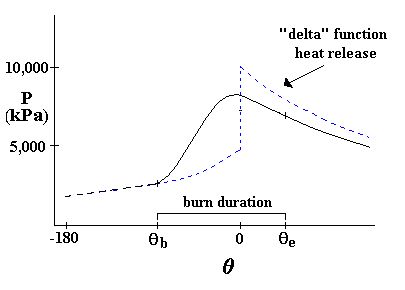Heat Release Model

Link to: Heat Release Fraction Applet

Link to: Simple Heat Release Applet

#### Derivation of Pressure versus Crank Angle for Finite Heat Release

The differential first law for this model for a small crank angle change,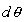, is:Using the following definitions,heat release ,and, results in :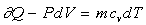The ideal gas equation is PV = mRT, soandThe first law now becomesFurther reducing the equation:Using R = cp-cv and k = cp/cv, to define, the energy equation after rearrangement becomes: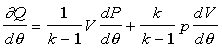or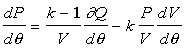If we know the pressure, P, volume, V,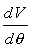, the heat released gradient,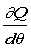, we can compute the change in pressure,. Thus explicitly solving the equation for pressure as a function of crank angle. Alternatively, we can use experimental data for the pressure, P and the volume, V, to determine the heat release term by solving for.

First, the volume, V and, have to be defined. From the slider-crank model, we have a definition for cylinder volume, V. Both terms are only dependent on engine geometry.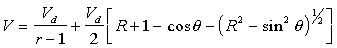So taking the derivative with respect to the crank angle,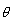, results in :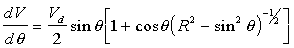For heat release term,, the Wiebe function for the burn fraction is used.Where:
f        = the fraction of heat added= the crank angle0  = angle of the start of the heat addition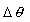= the duration of the heat addition (length of burn)
a       = usually 5
n       = usually 3

To view the burn fraction, f, as a function of the crank angle, click here: Heat Release Applet.

At the beginning of combustion, f = 0, and at the end the fraction is almost 1.

The heat release,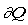, over the crank angle change,, is: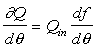Where Qin is the overall heat input.

Taking the derivative of the heat release function, f, with respect to crank angle, gives the following definition of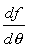.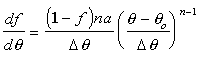If, dF = 0.

So now withanddefined, the pressure as a function of the crank angle can be solved.

The following applet plots the pressure, work and temperature as a function of the crank angle: Simple Heat Release Applet. The effect of heat transfer to the cylinder wall can also be included.